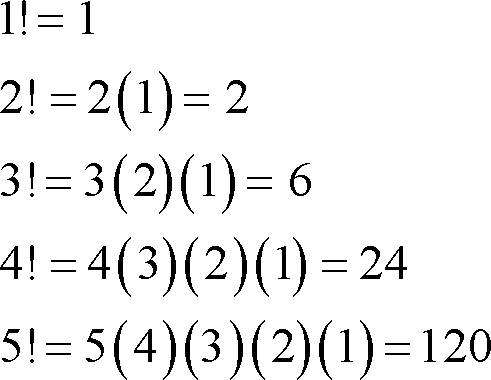# Factorial Program In Java – 5 Simple Ways | Java Tutoring

Factorial program in java. Here is the list of different types of factorial java code along with sample outputs. If you have no idea on how to solve the Factorial in math, do check out our tutorial below so that you will get an idea. The Factorial program in Java, we have written the following program in five different ways, using standard values, using while loop, using for loop, using do while loop, using method or function, using recursion.

If you have any doubts related to the code that we shared below do leave a comment here at the end of the post our team will help you out related to ant query.

Q > So, basically what is factorial?

Def: A factorial is a function that multiplies number by every number. For example 4!= 4*3*2*1=24. The function is used, among other things, to find the number of ways “n” objects can be arranged.

In mathematics, there are n! ( Factorial ways to arrange N Objects ) in sequence.

Q > How to calculate?

For example :

Consider :

• 2! = 2 x 1 = 2

The possibility of 2! is two ways like {2,1}, { 1,2 }.

Same as like :

4! = 4 x 3 x 2 x 1 = 24.

• 24 = the arrangement of 4! is {1,2,3,4}, {2,1,3,4}, {2,3,1,4}, {2,3,4,1}, {1,3,2,4}, etc.

Same as like 5! , 10! , N!.The following program has been written in 5 different ways, using while loop, for loop, do while loop, using method.

Now, let’s get into the programming part.

## Java Program To Calculate Factorial in 5 Different Ways

1. Java Program To Calculate Factorial using standard values with outputs

Standard values – consider the following code is universally applicable- with sample outputs.

output:

### 2. Java Program Using For Loop

• Using for loop: Here is the program using for loop with sample outputs #example.

Among all three loops, for loop is probably the most used loop. For loop are two types mainly:

1. for each style of for loop
2. normal for loop
output:

3. Using Command Line Arguments

Using command line arguments: Here is the complete guide about command line arguments in Java with examples.

output:

4. Using Function

Java code for calculating Factorial using a user-defined method(using function).

output:

#### 5. Using Recursion

Recursion: A Recursion is a function call itself – you can check out more information about what is recursion in java here?

output:

##### 6.Using while loop

Here is the code using the while loop: The definition of while loop is to execute the set of statements as long as the condition is true. Here we share the complete guide on what is while loop in java with sample examples and syntax – do check it out. If you have any doubts related to the following program using while loop, do let us know here.

output:
###### 7.Using Do While Loop

The difference between while loop and do while loop is, wherein do while the condition is checked in each iteration, whereas in while loop the condition is checked at the beginning of each iteration. Here is the complete guide on Java do while with examples and syntax.

output:
That’s it.

Also, check :

x

## X Star Pattern Java Program – Patterns

Java program to print X star pattern program – We have written the below print/draw ...